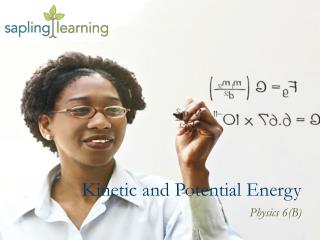DownloadDownload PresentationKinetic and Potential Energy

# Kinetic and Potential Energy

Download Presentation## Kinetic and Potential Energy

- - - - - - - - - - - - - - - - - - - - - - - - - - - E N D - - - - - - - - - - - - - - - - - - - - - - - - - - -
##### Presentation Transcript

1. Kinetic and Potential Energy Physics 6(B)

2. Learning Objectives • Explain the differences between kinetic and potential energy and their sources • Describe situations involving kinetic and potential energy • Explain how potential energy is transformed into kinetic energyand kinetic energy is transformed into potential energy • Kinetic and Potential Energy

3. Kinetic Energy(KE) – the energy of an object due to motion • Measured in joules, J • Dependent on: • Mass (m ) • Velocity (v) Defining Kinetic Energy

4. At the top, a skateboarder has no kinetic energy • Kinetic energy increases as the skateboarder moves downwards and speeds up • At the bottom, a skateboarder has maximum kinetic energy • Kinetic energy decreases as the skateboarder moves upwards and slows down • Kinetic Energy

5. Potential Energy(PE) – the energy of a system due to its position • Measured in joules, J • Dependent on: • Mass (m ) • Height above ground (h ) • Acceleration due to gravity (g ) Defining Potential Energy

6. On the ground, each block has no potential energy • On the stairs, the yellow object has the least potential energy and the green object has the most potential energy • Potential Energy

7. Energy can be transformed from one type to another • In the picture on the left, the ball’s potential energy is at a maximum and its kinetic energy is at a minimum • In the picture on the right, the ball’s kinetic energy is at a maximum and its potential energy is at a minimum • Energy Transformation

8. Transformation between kinetic and potential energy is never perfect • Energy can be “lost” when it is transformed out of a system • Some kinetic and potential energy of the roller coaster is transformed into heat and sound • Energy Transformation

9. Learning Objectives • Explain the differences between kinetic and potential energy and their sources • Describe situations involving kinetic and potential energy • Explain how potential energy is transformed into kinetic energy and kinetic energy is transformed into potential energy • Kinetic and Potential Energy